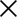Click here to view our Special offers for November and December.

# How do you measure the flange size?

When measuring a flange, the most important dimension is the PCD (Pitch Circle Diameter).  The PCD is the diameter of a circle through the centre of each hole.

When measure a valve or flange that is already installed, if there are no stampings on the flanges or valves here is a handy tip to calculate.

Assuming you can get to the bolts measure the centres of two adjacent bolts then use the following multipliers:

4 holes on flange multiply by 1.414

8 holes on flange multiply by 2.613

12 holes on flange multiply by 3.864

16 holes on flange multiply by 5.126

If you know the size or DN (Diameter Nominale) then check out our flange tables.